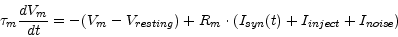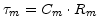LifNeuron
Subsections

## LifNeuron

### Model

A standard leaky-integrate-and-fire neuron model is implemented where the membrane potential Vm of a neuron is given bywhereis the membrane time constant, Rm is the membrane resistance, Isyn(t) is the current supplied by the synapses, Iinject is a non-specific background current and Inoise is a Gaussion random variable with zero mean and a given variance noise.

At time t=0 Vm ist set to Vinit . If Vm exceeds the threshold voltage Vthresh it is reset to Vreset and hold there for the length Trefract of the absolute refractory period.

### Implementation

The exponential Euler method is used for numerical integration.

Cm (F) :
The membrane capacity Cm
Rm (Ohm) :
The membrane resistance Rm
Vthresh (V) :
If Vm exceeds Vthresh a spike is emmited.
Vresting (V) :
The resting membrane voltage.
Vreset (V) :
The voltage to reset Vm to after a spike.
Vinit (V) :
The initial condition for Vm at time t=0.
Trefract (sec) :
The length of the absolute refractory period.
Inoise (A) :
The standard deviation of the noise to be added each integration time constant.
Iinject (A) :
A constant current to be injected into the LIF neuron.
type :
Type (e.g. inhibitory or excitatory) of the neuron